# Texas Go Math Grade 3 Lesson 16.2 Answer Key Use Area Models

Refer to our Texas Go Math Grade 3 Answer Key Pdf to score good marks in the exams. Test yourself by practicing the problems from Texas Go Math Grade 3 Lesson 16.2 Answer Key Use Area Models.

## Texas Go Math Grade 3 Lesson 16.2 Answer Key Use Area Models

Unlock the Problem

Melissa has a garden that is shaped like the rectangle below. Each unit square represents 1 square meter. What is the area of her garden?

Remember
A unit square can be different sizes: 1 square meter, 1 square foot, 1 square centimeter, and so on.

Count the number of rows.
Count the number of unit squares in each row.________ rows of _________ = ☐
So, the area is __________ square meters.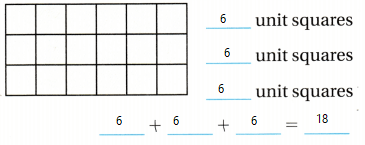Explanation:
3 rows of 6 = 18
So, the area is 18 square meters.

Another Way Use multiplication.

Count the number of rows. Count the number of unit squares in each row._________ rows of ________ = ☐
This figure is like an array. How do you find the total number of squares in an array?

Write a multiplication equation.
So, the area is _________ square meters.Explanation:
3 rows of 6= 18
3 x 6 = 18
So, the area is 18 square meters.

Math talk
Mathematical Processes

Explain when you can use different methods to the same area.
Explanation:
When we cannot count rows and columns

Share and Show

Question 1.
Find the area of the rectangle in two ways.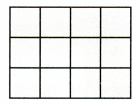_________ rows of _________ = ☐
Add. _________ + _________ + _________ = _________
Multiply. _________ × _________ = _________
What is the area of the rectangle?
_________ square units
3 rows of 4 = 12
Explanation:
The area of the rectangle in two ways
Add. 4 + 4 + 4 = 12
Multiply. 4 × 3 = 12
12 square units

Math talk
Mathematical Processes

Which method do you prefer using? Explain.
Explanation:
Because it is easy to find the area.

Find the area of the rectangle. Each unit square is 1 square foot.

Question 2.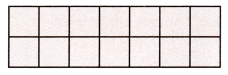2 x 7 = 14 square foot.
Explanation:
Number of rows x Number of Columns = Area

Question 3.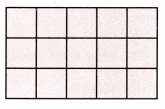3 x 5 = 15 square foot.
Explanation:
Number of rows x Number of Columns = Area

Find the area of the rectangle. Each unit square is 1 square meter.

Question 4.Answer:  4 x 8 = 32 square meter.
Explanation:
Number of rows x Number of Columns = Area

Question 5.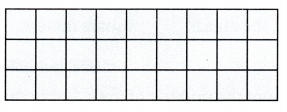Answer: 3 x 9 = 21 square meter.
Explanation:
Number of rows x Number of Columns = Area

Question 6.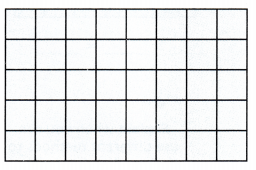Answer: 5 x 8 = 40 square meter.
Explanation:
Number of rows x Number of Columns = Area

Question 7.Answer: 5 x 5 = 25 square meter.
Explanation:
Number of rows x Number of Columns = Area

Problem Solving

Question 8.
Write Math Use Math Language Multi-Step Compare the areas of the two rugs at the right. Each unit square represents 1 square foot. Which rug has the greater area? Explain.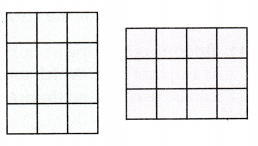Explanation:
Number of rows x Number of Columns = Area
4 x 3 = 12
3 x 4 = 12
Both the rugs areas are same
Question 9.
H.O.T. Pose a Problem A tile company tiled a wall using square tiles. A mural is painted in the center. The drawing shows the design. The area of each tile used is 1 square foot.Write a problem that can be solved by using the drawing. Then solve your problem.
Answer: What is the area of the mural
Explanation:
3 x 8 = 24
The total area is
5 x 10 = 50
50 – 24 = 26 is the boundaries.

Question 10.
H.O.T. Draw and shade three different rectangles with an area of 24 square units. Then write an addition or multiplication equation for each area.Explanation:
4 x 6 = 24
6 x 4 = 24
3 x 8 = 24

Fill in the bubble for the correct answer choice.

Question 11.
Brianna is coloring a bookmark. Each unit square is 1 square inch. Which multiplication equation can be used to find the area of the bookmark?(A) 7 + 7= 14
(B) 7 × 7 = 49
(C) 2 × 7 = 14
(D) 2 × 2 = 4
Explanation:
Brianna is coloring a bookmark. Each unit square is 1 square inch.
7 × 7 = 49 multiplication equation can be used to find the area of the bookmark

Question 12.
Maria is painting a tile design for her porch. Each square tile is 1 square foot. What is the area of the design?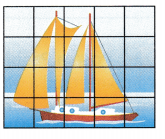(A) 9 square feet
(B) 18 square feet
(C) 16 square feet
(D) 20 square feet
Explanation:
Number of rows x Number of Columns = Area
4 x 5 = 20

Question 13.
Multi-Step Tom and Bill are painting a fence. Tom paints the yellow area. Bill paints the white area. Each unit square is 1 square foot. How many more square feet does Bill paint than Tom?(A) 10 square feet
(B) 40 square feet
(C) 15 square feet
(D) 25 square feet
Explanation:
5 x 3 = 15 is yellow
5 x 5 = 25 is white
25 – 15 = 10 more square feet does Bill paint than Tom

Texas Test Prep

Question 14.
Heather drew this rectangle. Which equation can be used to find the area of the rectangle?(A) 5 × 8 = 40
(B) 8 × 8 = 64
(C) 8 + 8 + 8 + 8 = 32
(D) 5 × 5 = 25
Explanation:
5 × 8 = 40 equation can be used to find the area of the rectangle

### Texas Go Math Grade 3 Lesson 16.2 Homework and Practice Answer Key

Find the area of the rectangle. Each unit square Is 1 square meter.

Question 1.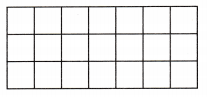Answer: 3 x 7 = 21 square meter.
Explanation:
Number of rows x Number of Columns = Area

Question 2.Answer: 4 x 6 = 24 square meter
Explanation:
Number of rows x Number of Columns = Area

Question 3.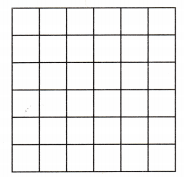Answer: 6 x 6 = 36 square meter
Explanation:
Number of rows x Number of Columns = Area

Question 4.Answer: 5 x 7 = 35 square meter
Explanation:
Number of rows x Number of Columns = Area

Problem Solving

Question 5.
Shoshauna is using one-inch tiles to cover a table. She wants to put a green border around the edge of the table. She wants to make the center of the table yellow. How many more yellow tiles does she need than green tiles? Explain.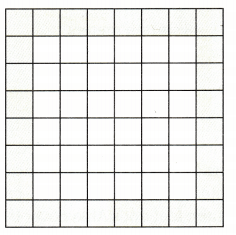Total area = 8 x 8 = 64
She wants to make the center of the table yellow
6 x 6  = 36 yellow
64 – 36 = 28 is green
36 – 28 = 8 more yellow tiles does she need than green tiles

Lesson Check

Question 6.
Nolan draws this diagram for a rug. Which equation can be used to find the area?(A) 5 + 8 = 40
(B) 5 × 5 = 25
(C) 8 × 5 = 40
(D) 8 × 8 = 64
Explanation:
Number of rows x Number of Columns = Area
8 x 5 = 40  equation can be used to find the area

Question 7.
Marisol puts together a floor puzzle. Each puzzle piece is 1 square foot. What is the area of the puzzle?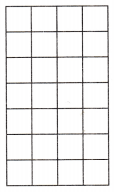(A) 27 square feet
(B) 28 square feet
(C) 32 square feet
(D) 25 square feet
Explanation:
The area of the puzzle is 28 square feet.

Question 8.
Multi-Step Lu Chen draws this diagram of his patio. Each square is 1 square meter. He wants to add 12 square meters to the patio. What will be the size of the new patio?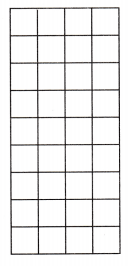(A) 40 square meters
(B) 36 square meters
(C) 42 square meters
(D) 48 square meters
Explanation:
Lu Chen draws this diagram of his patio.
Each square is 1 square meter. He wants to add 12 square meters to the patio.
48 square meters will be the size of the new patio
9 x 4 = 36 + 12 = 48

Question 9.
Multi-Step Aliki and Deke are covering a wall with panels. Aliki covers the yellow area. Deke covers the white area. Each panel is 1 square foot. How many more square feet does Aliki cover than Deke?(A) 6 square feet
(B) 8 square feet
(C) 9 square feet
(D) 7 square feet